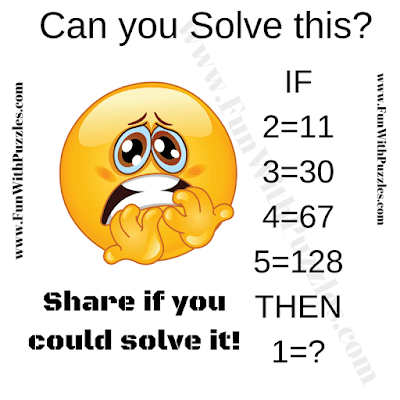Put your logical reasoning skills to the ultimate test with this intriguing Logical IQ Math Question! This brain teaser presents a series of logical equations that require your sharp wit to decipher the underlying logic. As you work your way through the given equations, your mission is to unravel the code that connects the numbers.

Once you've cracked the logic code, you'll be ready to tackle the final logical IQ puzzle! Can you confidently solve the last equation and find the missing number that will replace the question mark?

Get ready for an exciting journey of logical deduction and puzzle-solving prowess. Sharpen your brain and immerse yourself in the world of logical reasoning. Are you up for the challenge? Find out by solving this Logical IQ Math Question now!Can you solve this Logical IQ Math Question?

The answer to this "Logical IQ Math Question" can be viewed by clicking the button. Please do give your best try before looking at the answer.

Unknown said...

General knowledge said...

Good

Unknown said...

2^3+3=11
3^3+3=30
4^3+3=67
5^3+3=128
1^3+3=4